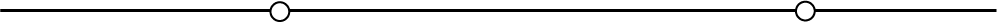## Extended Euclidean Plane

Geometry is founded by a set of basic elements and basic relations between them (which are not defined, but are intuitively clear) and a system of axioms (statements which are not proved, but are considered to be true and are intuitively obvious) from which other figures are defined and all corresponding consequences (theorems) are deduced.

In the plane geometry, which you have learned during your education in various ways (constructive and analytical), the basic elements are points and lines and the basic relation between them is the incidence relation*. This type of geometry was axiomatically developed by the greek mathematician Euclid in his treatise named Elements and is commonly known today as Euclidean plane geometry.
The constructions in this geometry are made using the ruler and compass.
The system of axioms, statements which are not meant to be proven but are intuitively clear (according to the Hilbert's axiomatics of the Euclidean plane there are 16 axioms), consists of five groups and includes measurement of length, angle and area. We shall state only two of these axioms:

A1: There exists exactly one line incident with two distinct points. (1st axiom of incidence)A5: Let p be a straight line and T a point not incident with p. Then there is at most one line that passes through T and does not intersect p. (axiom of parallels)• Two lines are parallel or they intersect each other in the Euclidean plane.

 Furthermore, the geometry of the projective plane also uses points and lines as the basic elements together with the basic relation, the relation of incidence. The system of axioms can be reduced to the following three axioms: A1: There exists exactly one line incident with two distinct points.A2: There exists exactly one point incident with two distinct lines .A3: There exist four distinct points of which neither three points are collinear. Therefore, there are no lines that do not intersect in the projective plane.Parallel tracks intersect in the picture.

We can describe projective geometry as the study of the geometric properties which are invariant under a central projection. The central projection is the closest to the image that develops in our eye and later it will be defined.

Note that the axioms A1 i A2 can be obtained from each other by the replacement of the words line and point. This fact conditions the principle of duality on the projective plane, i.e. if a statement is true in projective geometry it will remain true if we interchange the words line and point as well as the words join and intersect (lie on and pass through). Therefore, if we prove a theorem in the projective geometry, we also prove its dual statement.
Notions whose definitions are connected in such a way are referred to as dual notions. For example:
range of points (p) - a set of all points in the plane incident with a line p,
pencil of lines (P) - a set of all lines in the plane incident with a point P.

We extend the Euclidean plane in the following way:
• A point at infinity is added to every line and that point is the intersection point of each line parallel to the extended line. Different classes of parallel lines have different points at infinity.
• Points at infinity of all the lines in the plane lie on one line in the plane which we call the line at infinity.
The elements of the plane at infinity (line and points that lie on it) are real as are all the other elements of the plane.
The described Euclidean plane is called the extended Euclidean plane or real projective plane. In the projective plane every two lines intersect (parallel lines intersect at a point at infinity).

Projective geometry does not use measurements (the distance of points and measure of angles). Measure is a characteristic feature of the Euclidean geometry, and is indispensable in the field of engineering. Therefore we shall use the extended Euclidean plane (and later space) in our course for the deduction of certain general conclusions about the plane (spatial) figures, while we shall use the Euclidean plane (space) with the Euclidean metric for the constructions (on the paper or by computer).

* For the incidence relation we often use following expressions: "lie on" (point lies on the line), "pass through" (line passes through the point), "join" (line joins two points), "intersect" (two lines intersect) etc.
Points incident with the same line are called collinear and the lines incident with the same point are called concurrent.

Created by Sonja Gorjanc - 3DGeomTeh - Developing project of the University of Zagreb.
Translated by Helena Halas and Iva Kodrnja.